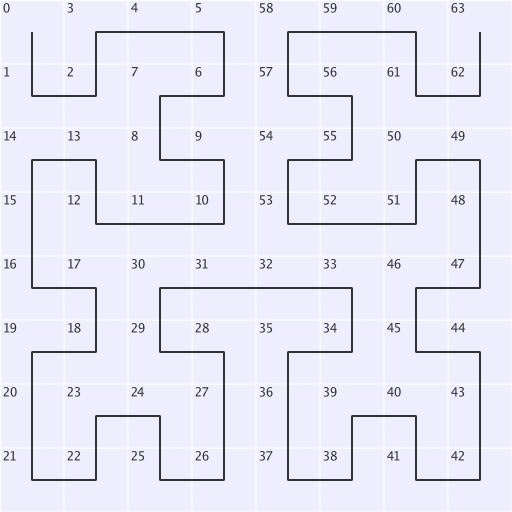# Introducing Hilbert. A Go library to map values onto a Hilbert curve.

| hilbert | go | opensource

A Hilbert curve is a space-filling (snakey) curve through a 2D space:Image of 8 by 8 Hilbert curve

This can be very useful for mapping a 1D value, into a 2D space. For example, it is commonly used to map IP addresses into a 2D space.

I recently created a library for Go that can map to and from a curve. The project is hosted on Github, and can be used like so:

``````import "github.com/google/hilbert"

// Create a Hilbert curve for mapping to and from a 16 by 16 space.
s, err := hilbert.New(16)

// Now map one dimension numbers in the range [0, N*N-1], to an x,y
// coordinate on the curve where both x and y are in the range [0, N-1].
x, y, err := s.Map(t)

// Also map back from (x,y) to t.
t, err := s.MapInverse(x, y)``````

The project contains some demos, such as this cool animations: